## Vehicle load limit тАУ how to determine

The load capacity of your vehicle is determined by weight, not by available cargo space. The load limit of your vehicle is shown on the vehicle placard attached to the driverтАЩs side B-pillar. Locate the statement тАЬThe combined weight of occupants and cargo should never exceed XXX kg or XXX lbsтАЭ on your vehicleтАЩs placard.

The vehicle placard also shows seating capacity of your vehicle.

The total load capacity includes the total weight of driver and all passengers and their belongings, any cargo, any optional equipment such as a trailer hitch, roof rack or bike carrier, etc., and the tongue load of a trailer. Therefore cargo capacity can be calculated by the following method.

For information about towing capacity and weight limits, refer to тАЬTrailer towingтАЭ .

Calculating total and load capacities varying seating configurations

Calculate the available load capacity as shown in the following examples:

Example 1AVehicle capacity weight of the vehicle is 900 lbs (408 kg), which is indicated on the vehicle placard with the statement тАЬThe combined weight of occupants and cargo should never exceed 900 lbs or 408 kgтАЭ.

For example, if the vehicle has one occupant weighing 154 lbs (70 kg) plus cargo weighing 662 lbs (300 kg).

1. Calculate the total weight.2. Calculate the available load capacity by subtracting the total weight from the vehicle capacity weight of 900 lbs (408 kg).3. The result of step 2 shows that a further 84 lbs (38 kg) of cargo can be carried.

Example 1BFor example, if a person weighing 176 lbs (80 kg) now enters the same vehicle (bringing the number of occupants to two), the calculations are as follows.

1. Calculate the total weight.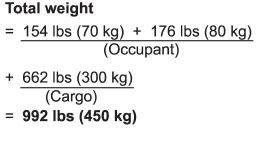2. Calculate the available load capacity.3. The total weight now exceeds the capacity weight by 92 lbs (42 kg), so the cargo weight must be reduced by 92 lbs (42 kg) or more.

Example 2AVehicle capacity weight of the vehicle is 900 lbs (408 kg), which is indicated on the vehicle placard with the statement тАЬThe combined weight of occupants and cargo should never exceed 408 kg or 900 lbsтАЭ.

For example, the vehicle has one occupant weighing 165 lbs (75 kg) plus cargo weighing 375 lbs (170 kg). In addition, the vehicle is fitted with a trailer hitch weighing 22 lbs (10 kg), to which is attached a trailer weighing 1,764 lbs (800 kg).

10% of the trailer weight is applied to the trailer tongue (i.e. Tongue load = 176 lbs (80 kg)).

1. Calculate the total weight.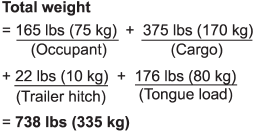2. Calculate the available load capacity.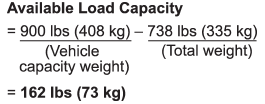3. The result of step 2 shows that a further 162 lbs (73 kg) of cargo can be carried.

Example 2BFor example, if a person weighing 143 lbs (65 kg) and a child weighing 40 lbs (18 kg) now enter the same vehicle (bringing the number of occupants to three), and a child restraint system weighing 11 lbs (5 kg) is installed in the vehicle for the child to use, the calculations are as follows.

1. Calculate the total weight.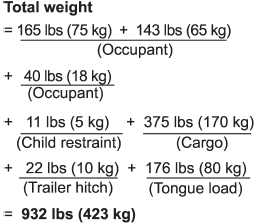2. Calculate the available load capacity.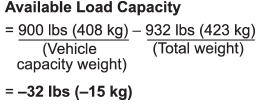3. The total weight now exceeds the capacity weight by 32 lbs (15 kg), so the cargo weight must be reduced by 32 lbs (15 kg) or more.